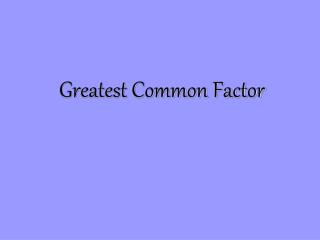DownloadDownload PresentationGreatest Common Factor

# Greatest Common Factor

Télécharger la présentation## Greatest Common Factor

- - - - - - - - - - - - - - - - - - - - - - - - - - - E N D - - - - - - - - - - - - - - - - - - - - - - - - - - -
##### Presentation Transcript

1. Greatest Common Factor

2. Greatest Common Factor What is aGreatest Common Factor? The greatest common factor (GCF) of a group of numbers is the largest (greatest)of all the factors the numbers have in common. Example: What is greatest common factor of the following group? 8, 12, 20 Though they have factors of 1, 2 and 4 in common,4 is the GCF

3. Greatest Common Factor When would we need to knowwhat the GCF of a group of numbers is? There are many times we want to know the GCF of a group of numbers. Here are a few: Reducing Fractions You can completely reduce a fraction in 1 stepif you divide the numerator and denominatorby the greatest of their common factors – the GCF!

4. Greatest Common Factor When would we need to knowwhat the GCF of a group of numbers is? There are many times we want to know the GCF of a group of numbers. Here are a few: Equal Divisions Say you have 3 pieces of wood…their sizes are:24 ft. 12 ft. 9 ft.You want to cut the wood into smaller pieces of the same size, but you want the pieces to be a big as possible, without any waste. How big should you cut them?

5. Greatest Common Factor How do you find a GCF? There are a number of ways to find a GCF of a group of numbers. Method 1: List all the factors of each number, and pick out the greatest of all the factors they have in common. Example: Find the GCF of 9, 12, 24 9: 1, 3, 9 12: 1, 2, 3, 4, 6, 12 24: 1, 2, 3, 4, 6, 8, 12, 24 They all have factors of 1 and 3. 3 would be the GCF.

6. Greatest Common Factor How do you find a GCF? There are a number of ways to find a GCF of a group of numbers. Method 2: The second method of finding the GCF of a group of numbers is to use their Prime Factorization. The GCF of a group of numbers isthe product of ALL their common prime factors.

7. Greatest Common Factor Finding the GCF using the Prime Factorization. The GCF of a group of numbers isthe product of ALL their common prime factors. Example: Find the GCF of: 24, 36, 60 1. We need the prime factorizations

8. Greatest Common Factor Finding the GCF using the Prime Factorization. The GCF of a group of numbers isthe product of ALL their common prime factors. Example: Find the GCF of: 24, 36, 60 2. Now we need to multiply all the common prime factors What do they all have in common? They all have two 2s in common.They all also have one 3 in common.

9. Greatest Common Factor Which method always works? BOTH! Which method is better? Depends on the numbers… With small numbers, listing the factors is probably easier With larger numbers,using the prime factorization is probably easiest

10. Greatest Common Factor Let's Practice… Use the prime factorization to find the GCF of the following group of numbers. 48, 72

11. Greatest Common Factor Let's Practice… Use the prime factorization to find the GCF of the following group of numbers. 48, 72

12. Greatest Common Factor Let's Practice… Use the prime factorization to find the GCF of the following group of numbers. 27, 64

13. Greatest Common Factor Let's Practice… Use the prime factorization to find the GCF of the following group of numbers. 27, 64

14. Greatest Common Factor Special Groups of Numbers If the GCF of a group of numbersis 1, then we call the group… RELATIVELY PRIME A group of numbers is "relatively prime"if they have no common factors other than 1. Can you think of a group of numbers thatwould be considered relatively prime?

15. Greatest Common Factor Which group(s) of numbers are relatively prime? 4, 12, 20 3, 9, 18, 23 9, 72 2, 3, 4

16. Greatest Common Factor More Practice… Use the prime factorization to find the GCF of the following group of numbers. 128 & 96 360 & 540